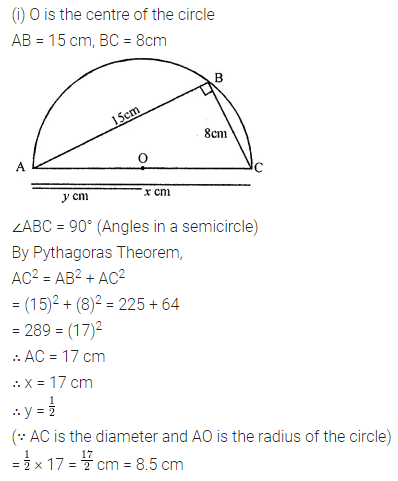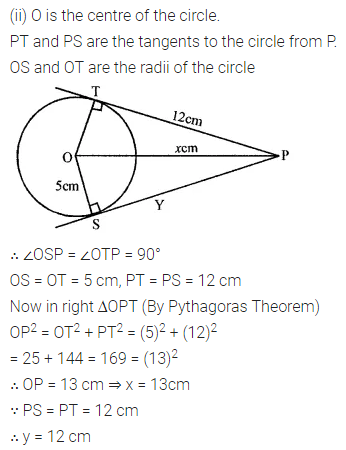## ML Aggarwal Class 8 Solutions for ICSE Maths Chapter 15 Circle

Question 1.
Draw a circle with centre O and radius 2.5 cm. Draw two radii OA and OB such that ∠ AOB = 60°. Measure the length of the chord AB.
Solution: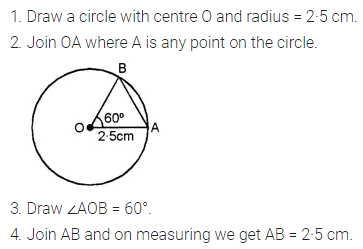Question 2.
Draw a circle of radius 3·2 cm. Draw a chord AB of this circle such that AB = 5 cm. Shade the minor segment of the circle.
Solution: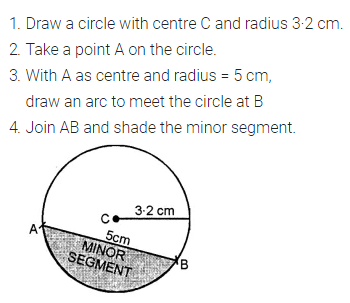Question 3.
Find the length of the tangent drawn to a circle of radius 3 cm, from a point at a distance 5 cm from the centre.
Solution:Question 4.
In the adjoining figure, PT is a tangent to the circle with centre C. Given CP = 20 cm and PT = 16 cm, find the radius of the circle.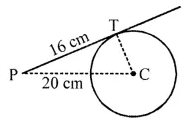Solution: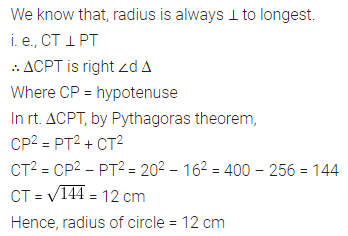Question 5.
In each of the following figure, O is the centre of the circle. Find the size of each lettered angle :Solution: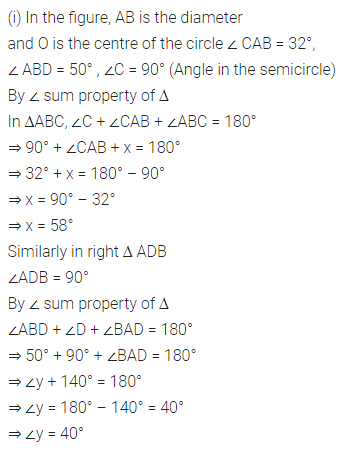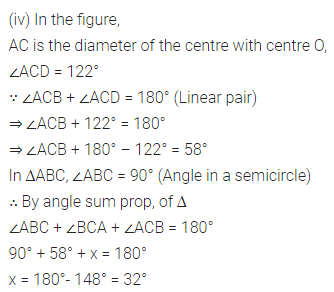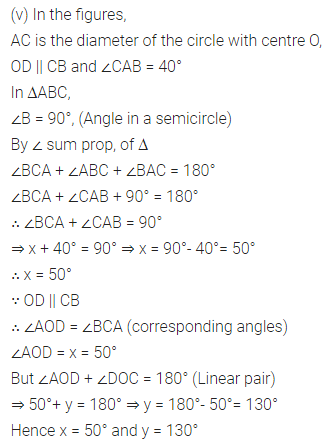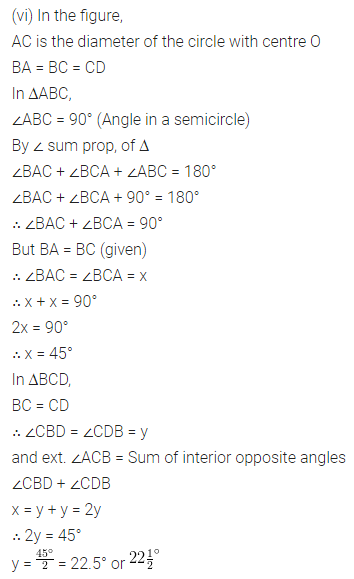Question 6.
In each of the following figures, O is the j centre of the circle. Find the values of x and y.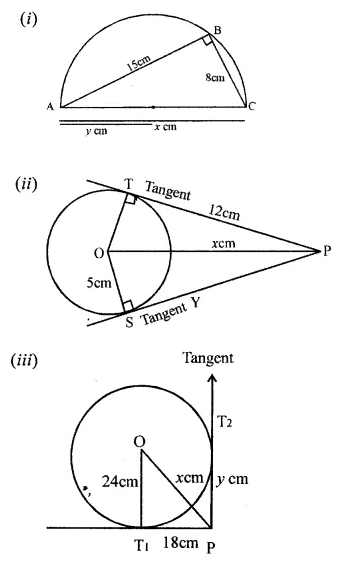Solution: